Author: Oscar Cronquist Article last updated on February 03, 2020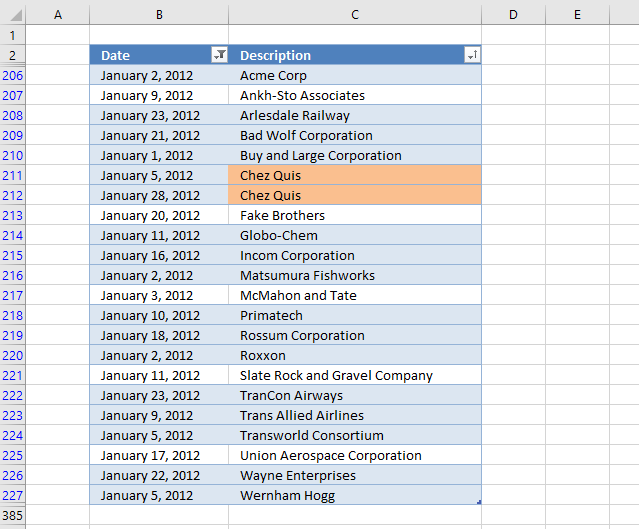The image above demonstrates a conditional formatting formula applied to an Excel Table containing random data. The Excel Table has been filtered to show only records for January 2012.

There is a built-in function in Excel that lets you highlight duplicates, however, that won't work properly if you have filtered the data.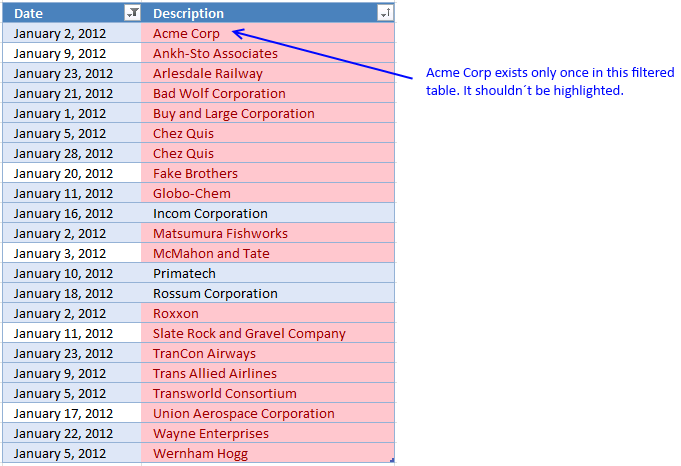The image above shows the built-in tool highlighting duplicate items. But one item exists only once in the filtered data, however, it is highlighted as a duplicate.

The reason is that there is another item not showing in the filtered table. You need to rely on a custom conditional formatting formula if you want it to compare only filtered visible values.

Note, Conditional formatting is volatile and may slow down your worksheet considerably.

### Build an Excel Table

The reason I am using an Excel Table is that the conditional formatting adjusts automatically if you add or delete records. It also uses structured references so there is no need to change cell references in the formula.

The disadvantage is that you have to use the INDIRECT function each time you reference the Excel Table in the CF formula, it is not a big deal but it is worth noting.

1. Select any cell in your data set.
2. Press short cut keys CTRL + T to open the "Create Table" dialog box.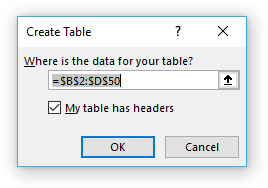3. Enable the checkbox if the columns in the data set have header names.
4. Press with left mouse button on OK button to apply.

### Create a new conditional formatting rule

1. Select table column "Description".
2. Go to the "Home" tab on the ribbon.
3. Press with left mouse button on the "Conditional formatting" button.
4. Press with left mouse button on "New Rule..".
5. Press with left mouse button on "Use a formula to determine which cells to format".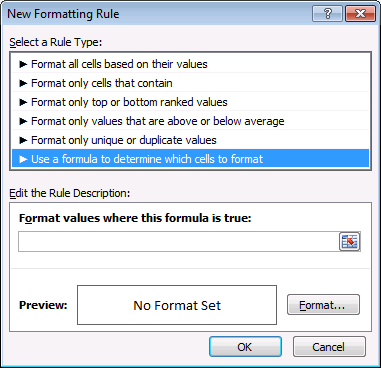6. Copy this conditional formatting formula:
=SUM(COUNTIF(INDIRECT("Table2[@Description]"), IF(SUBTOTAL(3, OFFSET(INDIRECT("Table2[Description]"), MATCH(ROW(INDIRECT("Table2[Description]")), ROW(INDIRECT("Table2[Description]")))-1, 0, 1)), INDIRECT("Table2[Description]"), "")))>1
7. Paste to "Format values where this formula is true:". I will explain the formula later in this article.
8. Press with left mouse button on the "Format..." button.
9. Go to tab "Fill".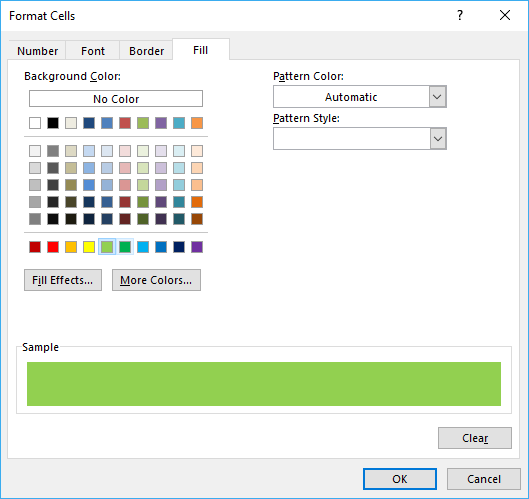10. Pick a color.
11. Press with left mouse button on OK button.
12. Press with left mouse button on OK button again.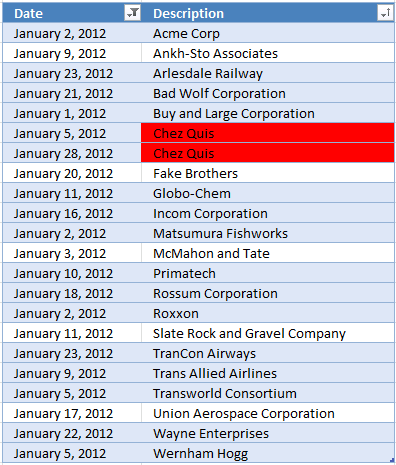### Explaining CF formula in cell C211

This Conditional Formatting formula highlights cells that have a duplicate in a filtered Excel Table, note that it will not be highlighted if a duplicate exists outside the filtered values (not visible).

#### Step 1 - Create an array from 1 to n

The INDIRECT function makes it possible to use a reference to an Excel Table inside a Conditional Formatting formula. This article explains it in greater detail: How to use an Excel Table name in Data Validation Lists and Conditional Formatting formulas

The ROW function converts a cell reference to the corresponding row numbers which is useful when you want to create an array.

The MATCH function is utilized in this example to create an array that contains a sequence that begins with 1 and increments with one up to the number of values in the array.

MATCH(ROW(INDIRECT("Table2[Description]")), ROW(INDIRECT("Table2[Description]"))

becomes

MATCH(ROW(C206:C227), ROW(C206:C227))

becomes

MATCH({3; 4; ... 383; 384}, {3; 4; ... 383; 384})

and returns

{1; 2; 3; ... 380; 381}.

The Excel Table has 381 records and the size of the array matches that number.

#### Step 2 - Modify array

This workaround makes it possible to use an array of values in a SUBTOTAL function, the OFFSET function splits the array into smaller arrays with only one value in each array.

OFFSET(reference,rows,columns,[height],[width])

It returns error values, however, the SUBTOTAL function can calculate these values anyway.

OFFSET(INDIRECT("Table2[Description]"), MATCH(ROW(INDIRECT("Table2[Description]")), ROW(INDIRECT("Table2[Description]")))-1, 0, 1)

becomes

OFFSET(INDIRECT("Table2[Description]"), {1; 2; 3; ... 380; 381}-1, 0, 1)

becomes

{0; 1; 2; ... ; 379; 380}

and returns

{#VALUE!; #VALUE!; #VALUE!; ... ; #VALUE!; #VALUE!}

#### Step 3 - Identify visible values

The first argument in the SUBTOTAL function is 3 and represents the COUNTA function meaning it will count cells that are not empty.

The SUBTOTAL function will actually return an array with this setup which is very handy in this situation.

SUBTOTAL(3, OFFSET(INDIRECT("Table2[Description]"), MATCH(ROW(INDIRECT("Table2[Description]")), ROW(INDIRECT("Table2[Description]")))-1, 0, 1))

becomes

SUBTOTAL(3, {#VALUE!; #VALUE!; #VALUE!; ... ; #VALUE!; #VALUE!})

and returns {0; 0; 0; ... ; 0; 0}. 0 (zero) represents a hidden value and 1 is visible.

#### Step 4 - Create an array containing visible values

The IF function replaces 0 (zero) with nothing "" and 1 with the actual corresponding value.

IF(SUBTOTAL(3, OFFSET(INDIRECT("Table2[Description]"), MATCH(ROW(INDIRECT("Table2[Description]")), ROW(INDIRECT("Table2[Description]")))-1, 0, 1)), INDIRECT("Table2[Description]"), "")

becomes

IF({0; 0; 0; ... ; 0; 0}, INDIRECT("Table2[Description]"), "")

and returns {""; ""; ""; ... ; ""; ""}.

#### Step 5 - Check the number of times the current value exists across visible values

The COUNTIF function will return an array with this setup which we then can use to calculate a total. The reason we don't change the arguments with each other is that the range argument will not accept an array based on calculations.

COUNTIF(range, criteria)

COUNTIF(INDIRECT("Table2[@Description]"), IF(SUBTOTAL(3, OFFSET(INDIRECT("Table2[Description]"), MATCH(ROW(INDIRECT("Table2[Description]")), ROW(INDIRECT("Table2[Description]")))-1, 0, 1)), INDIRECT("Table2[Description]"), ""))

becomes

COUNTIF(INDIRECT("Table2[@Description]"), {""; ""; ""; ... ; ""; ""})

becomes

COUNTIF("Chez Quiz", {""; ""; ""; ... ; ""; ""})

and returns {0; 0; 0; ... ; 0; 0}. The entire array is not shown, however, it contains a few 1's as well.

#### Step 6 - Calculate a total

The SUM function adds all numbers in the array and returns a total.

SUM(COUNTIF(INDIRECT("Table2[@Description]"), IF(SUBTOTAL(3, OFFSET(INDIRECT("Table2[Description]"), MATCH(ROW(INDIRECT("Table2[Description]")), ROW(INDIRECT("Table2[Description]")))-1, 0, 1)), INDIRECT("Table2[Description]"), "")))

becomes

SUM({0; 0; 0; ... ; 0; 0})

and returns 2.

#### Step 7 - Check if value is larger than 1

The > larger than character is a logical operator that lets you compare values, it will return a boolean value TRUE or FALSE based on the outcome. The Conditional Formatting formula uses the boolean function to determine if the cell values should be highlighted or not.

SUM(COUNTIF(INDIRECT("Table2[@Description]"), IF(SUBTOTAL(3, OFFSET(INDIRECT("Table2[Description]"), MATCH(ROW(INDIRECT("Table2[Description]")), ROW(INDIRECT("Table2[Description]")))-1, 0, 1)), INDIRECT("Table2[Description]"), "")))>1

becomes

2>1

and returns True. Cell C211 is highlighted.

### Get the Excel fileHighlight-duplicates-in-a-filtered-table.xlsx